Constructing a triangle when 2 sides and angle between is known (SAS)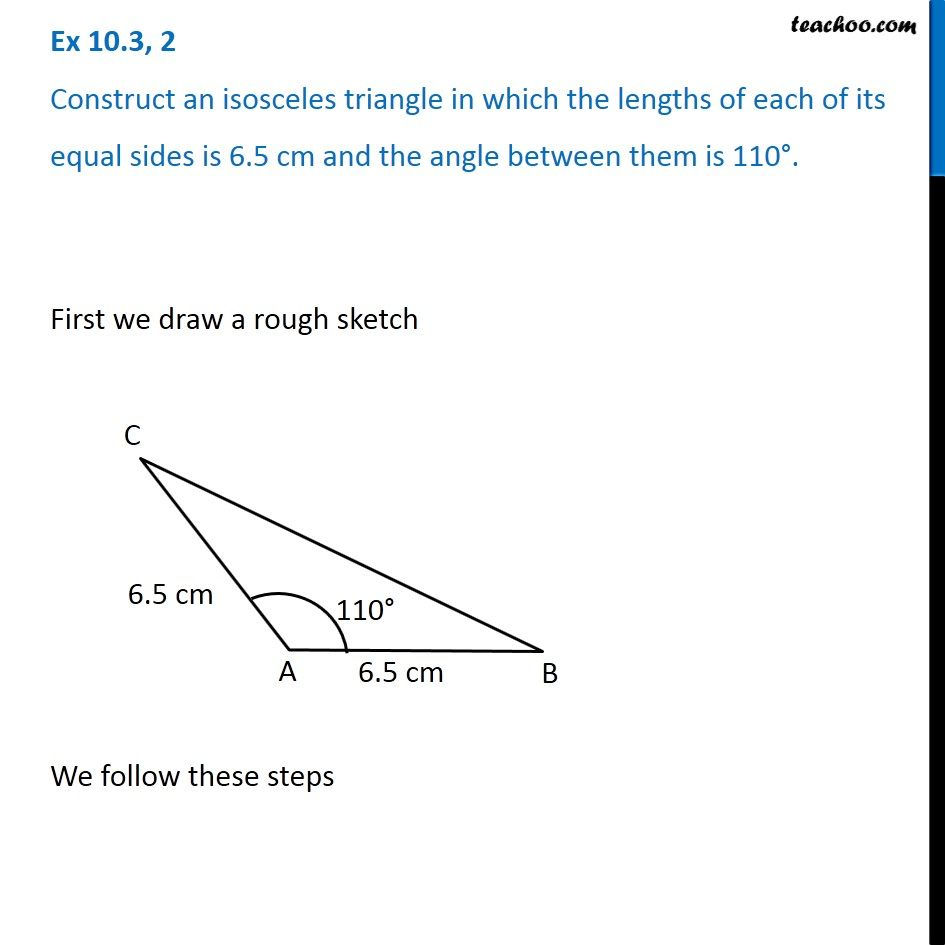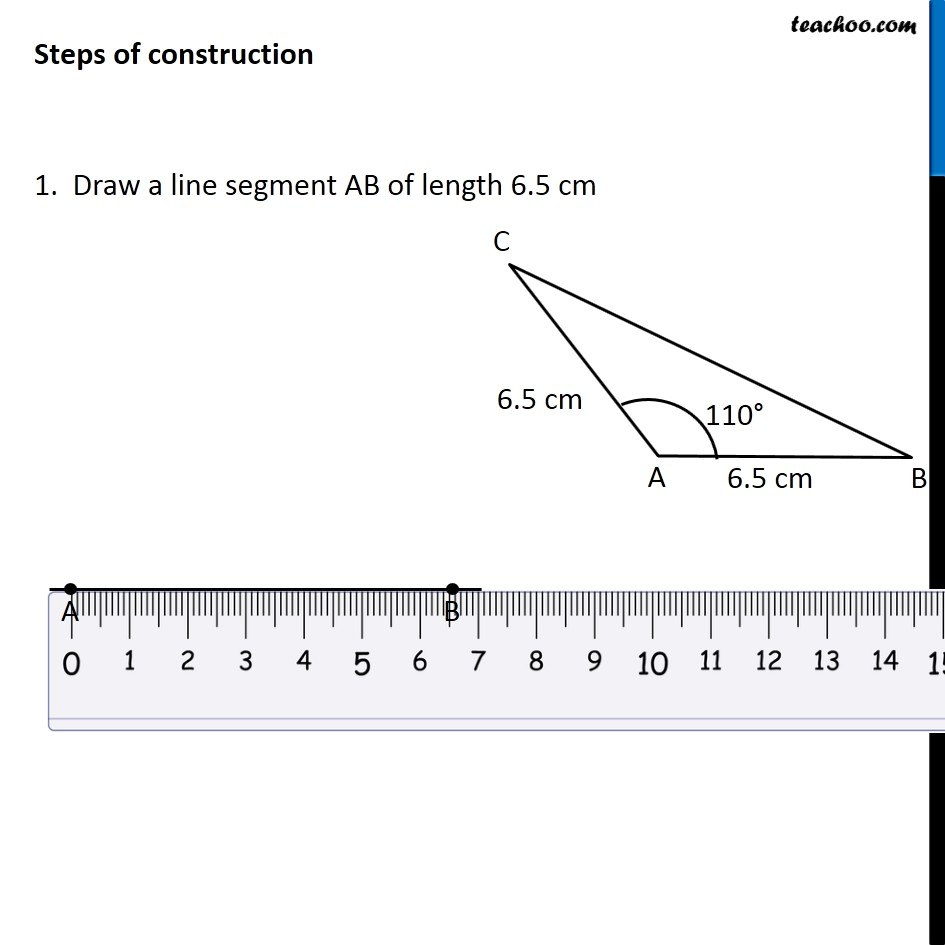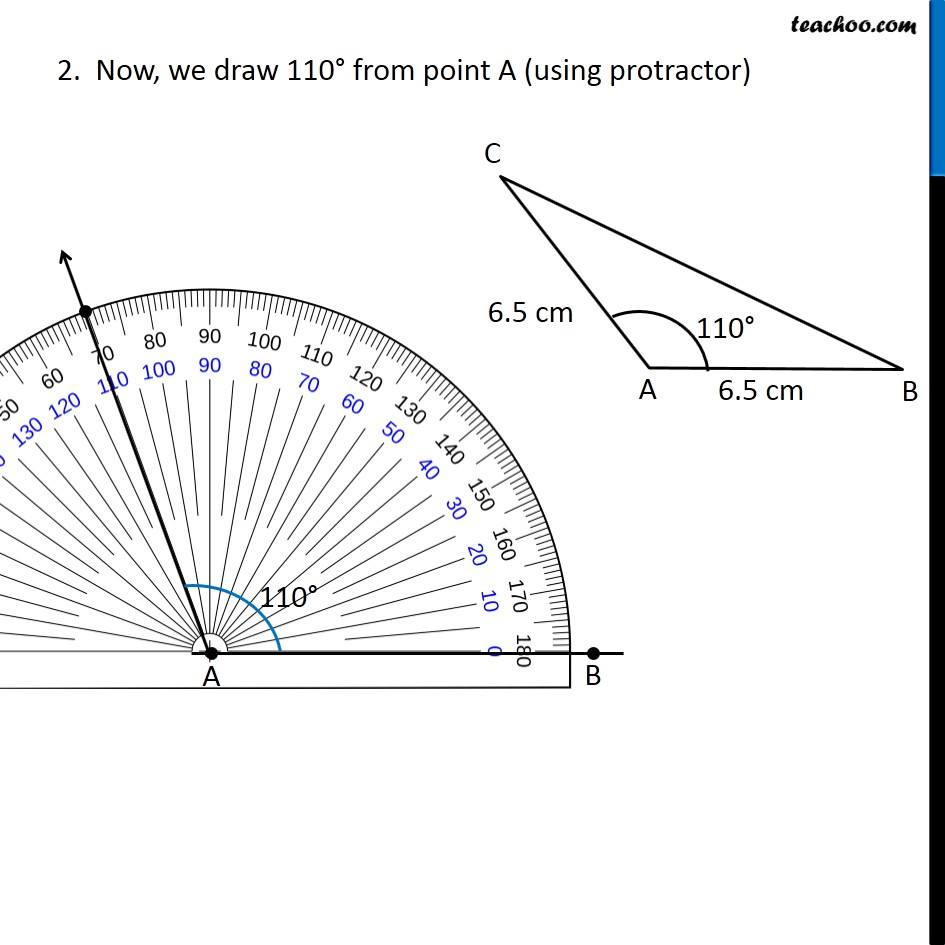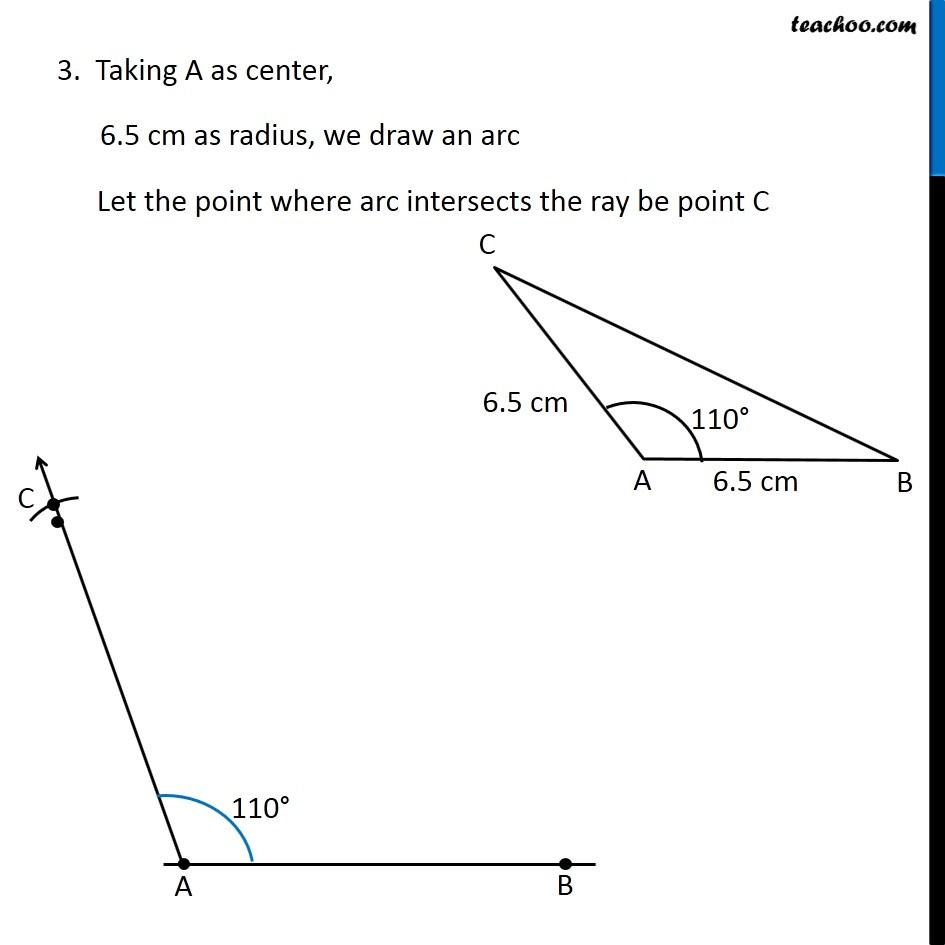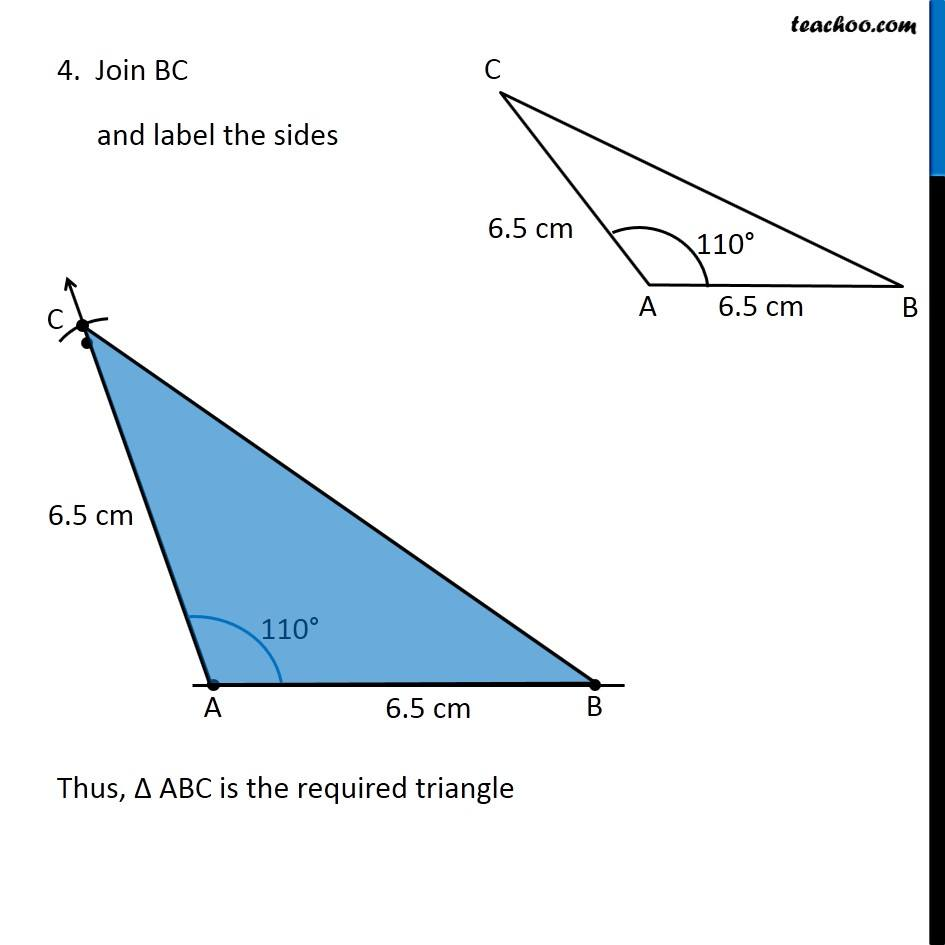Introducing your new favourite teacher - Teachoo Black, at only ₹83 per month

### Transcript

Ex 10.3, 2 Construct an isosceles triangle in which the lengths of each of its equal sides is 6.5 cm and the angle between them is 110°. 2. Now, we draw 110° from point A (using protractor) 3. Taking A as center, 6.5 cm as radius, we draw an arc Let the point where arc intersects the ray be point C 4. Join BC and label the sides Thus, Δ ABC is the required triangle